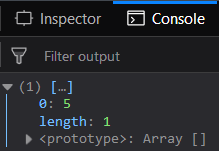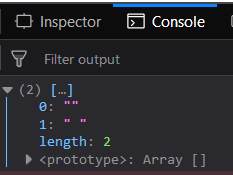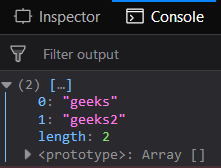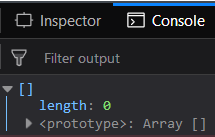Open in App
Not now

# Underscore.js _.difference() Function

• Last Updated : 24 Nov, 2021

The Underscore.js is a JavaScript library that provides a lot of useful functions like the map, filter, invoke etc even without using any built-in objects.
The _.difference() function returns the values of array that are not present in the other arrays. If element is present in array then this element will not be included in the resultant array. This is because it takes difference of the second array and first array.

Syntax:

`_.difference( array, *others )`

Parameters: This function accepts two parameters as mentioned above and described below:

• array: This parameter is used to hold the array elements.
• others: It is an array whose elements need to be deleted.

Return value: This function returns an array which contains elements of the first array which are not in the second array.

Passing a list of numbers to _.difference() function: The ._difference() function takes the element from the list one by one and checks if that element is present in the second array or not. If it is present then it simply ignores the element otherwise add the elements in the resultant array.

Example:

 ```<``html``>``    ``<``head``>``        ``<``script` `src` `= ``"https://cdnjs.cloudflare.com/ajax/libs/underscore.js/1.9.1/underscore-min.js"` `>``        ````    ````    ``<``body``>``        ``<``script` `type``=``"text/javascript"``>``            ``console.log(_.difference([1, 2, 3, 4, 5], ``                                     ``[1, 2, 3, 4, 6, 7]));``        ````    ````                    `

Output:Passing false values to the _.difference() function: Passing the false values like null, undefined, false, “” (empty string) then the _.difference() function will work in the same way. If any of the false values are present in the first array but not present in the second array then it will be included in the resultant array.

Example:

 ```<``html``>``    ``<``head``>``        ``<``script` `src` `= ``"https://cdnjs.cloudflare.com/ajax/libs/underscore.js/1.9.1/underscore-min.js"` `>``        ````    ````    ``<``body``>``        ``<``script` `type``=``"text/javascript"``>``            ``console.log(_.difference([undefined, '', " ", null], ``                                     ``[undefined, 4, null]));``        ````    ````                    `

Output:Passing a set of strings to the _.difference() function: Passing the set of strings which contains words inside “” then the _.difference() function will work in the same way. If any of the these string values are present in the first array but not present in the second array then it will be included in the resultant array.

Example:

 ```<``html``>``    ``<``head``>``        ``<``script` `src` `= ``"https://cdnjs.cloudflare.com/ajax/libs/underscore.js/1.9.1/underscore-min.js"` `>``        ````    ````    ``<``body``>``        ``<``script` `type``=``"text/javascript"``>``            ``console.log(_.difference(``                ``["This", "is", "geeks", "for", "geeks2"], ``                ``["This", "for", "is"])``            ``);``        ````    ````                    `

Output:Passing two arrays which have the same elements to the _.difference() function: Passing the first and the second array having the same elements then all the elements during the check operation will be ignored. And hence the resultant array will be empty.

Example:

 ```<``html``>``    ``<``head``>``        ``<``script` `src` `= ``"https://cdnjs.cloudflare.com/ajax/libs/underscore.js/1.9.1/underscore-min.js"` `>``        ````    ````    ``<``body``>``        ``<``script` `type``=``"text/javascript"``>``            ``console.log(_.difference([1, 2, 3, 4], [1, 2, 3, 4]));``        ````    ````                    `

Output:Note: These commands will not work in Google console or in Firefox as for these additional files need to be added which they didn’t have added. So, add the given links to your HTML file and then run them.

 `<``script` `type``=``"text/javascript"` `src` `= ``"https://cdnjs.cloudflare.com/ajax/libs/underscore.js/1.9.1/underscore-min.js"``> `` `

My Personal Notes arrow_drop_up